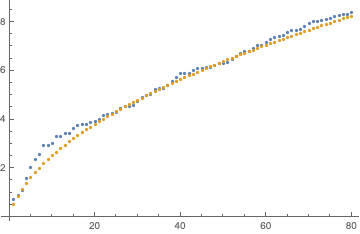# On partial sum estimate on the series $S(p,q;s)=\sum_1^q\frac{\sin^2(\frac{p\Gamma(n)}{n})}{n^s}$ and other Generalizations

Consider the following sum :

$$S(p,q;s)=\sum_{n=1}^q\frac{\sin^2(\frac{p\Gamma(n)}{n})}{n^s}$$

Here , $$p$$ is a variable w.r.t which we are going to analyse the sum.

$$s$$ is another parameter with domain $$s\in(0,1]$$.

I tried to use Abel - Plana summation formula (ABSF) for partial sum :

we can get some crude estimates on integral :

$$I_1(q)= \int_1^q\frac{\sin^2(\frac{p\Gamma(t)}{t})}{t^s}dt$$

as $$q\rightarrow \infty$$

( Integrand oscillates very wildly in right half plane )

But it seems that second integral in ABSF is impregnable .

Second integral in ABSF:

$$I_2(x)=\int_0^\infty \frac{F(x + iy,s) − F(x − iy,s)}{e^{2πy}-1}dy$$

Where , $$F(z)=\frac{\sin^2(\frac{p\Gamma(z)}{z})}{z^s}$$

I tried to get estimate on this as $$x\rightarrow \infty$$ but in vain .

The reason I chose ABSF is that $$\Gamma$$ is a 'nice' function in terms of complex variables and due to this I'm optimistic about the $$I_2$$

The importance of this function lies in the fact that , See for ex . for $$p=π/2$$ the $$\sin^2$$ term is finite for primes and zero for non primes . So I mentioned 'sharp' for this purpose . I need critical details of $$I_2$$.

( I'm just following the advice of F.R.Villegas to generalize the series with such parametrization.)

Question : Can we get an 'Sharp' estimates on the sum w.r.t parameters $$p$$ and $$s$$?

Can we prove the divergence of series (as a whole) for specified conditions on $$p$$?

(Also , I calculated various values of $$I_2(x)$$ for various $$x$$'s and test parameters .)

Edit:

More generally we can consider the following:

$$F(z) = \omega(z)\sin^2\left(\frac{π\Gamma(z)}{2z}\right)$$

Here, $$\omega(z)$$ is a weight we have to construct.

I try to construct the $$\omega(z)$$ s.t.

$$\sum_2^n F(n)= \int_2^n F(x)dx + A$$

Here A is constant.

For this to be true the following three conditions should meet for $$\omega(z)$$ in context of APSF:

1. $$\omega(z)>\frac{1}{z},\ \forall z\in\mathbf{R}$$

( More generally this condition is added for divergence of $$\int_c^\infty F(x)dx$$ So , $$\omega(z)$$ can even be complex valued for real domain as long as the given integral is divergent )

1. $$\lim_{ y→∞}|F(x ± iy)|e^{−2πy }= 0$$

2. $$\int_0^\infty |F(x + iy) − F(x − iy)|e^{−2πy} dy<+\infty$$ for every $$x≥1$$ and tends to zero as $$x\to\infty$$.

Can we Explicitly construct $$\omega(z)$$?

Even if one can omit 1st condition and able to construct the weight s.t. it follows condition 2,3 please mention. ( i.e in this case the integral $$\int_2^\infty F(x)dx$$ is convergent.

Also one can generalize further:

$$F(z) = {\phi(\sin^2[π\Gamma(z)/(2z)])}$$

S.t.

(1)$$ϕ(x)=0$$ if x is zero ; and 'suitably' finite otherwise (Here , 'suitably' means a value which guarantees the expected divergence of sum (very close to 1 or greater than or equal to 1) )

(2) condition (3) holds for such function.

Could we make above analysis workable?

Also, I think the condition (3) is very hard to achieve because of the complex roots of equations

$$\Gamma(z)/(z)$$=even integer

Also if condition (3) is not possible in any way, can we get estimate on analogues $$I_2(x)$$ with use of suitable weight which makes things easier?

If this could be achieved then we are able to get the estimates on primes using purely analytic information ( no number theoretic information like Euler product ) . And this seems (although extremely hard but,) possible as due to relatively elementary nature of summand and integrals .

Note: I know this question received negative reviews (due to both my behavior and insufficient information ) but please consider the importance of question.

• I would think this is divergent for any $s\in(0,1]$. Feb 24, 2020 at 19:00
• Thank you everyone who upvoted! At least question now maintain a zero score. Apr 11, 2021 at 8:16

I would just approximate $$\sin^2$$ by 1/2, it oscillates rapidly and the average over one period should be a sensible estimate, then $$S(p,q,s)\approx \tfrac{1}{2}H_q^{(s)},$$ the Harmonic number. For large $$q$$ and $$s<1$$ this gives $$S(p,q,s)\rightarrow q^{-s} \left(\frac{q}{2 (1-s)}+\tfrac{1}{4}+{\cal O}(q^{-2})\right)+\tfrac{1}{2}\zeta (s),$$ while for $$s=1$$ one has $$S(p,q,1)\rightarrow=\tfrac{1}{2} \ln q+\tfrac{1}{2}\gamma_{\rm Euler}+\frac{1}{4 q}+{\cal O}(q^{-2}).$$ Here is a comparison for $$p=1$$, $$s=1/2$$ (blue the exact sum, orange the large $$q$$ asymptotics).• thank you for the answer. But, the main purpose of the question is very different . See for ex . for $p=\pi/2$ the $\sin²$ term is finite for primes and zero for non prime . So I mentioned 'sharp' for this purpose . I need critical details of $I_2$. (The sum is very delicate for such critical values )I'm just following the advice of F.R.Villegas to generalize the series with such parametrization Feb 24, 2020 at 20:03
• OK, this was not clear at all to me from the question, apologies, the estimate I gave in this answer applies to generic values of $p$; so you want to be able to estimate something like $\sum_{k=\text{prime}}k^{-s}\cos^2(\pi/2k)$. Feb 24, 2020 at 20:20